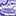# QlikView App Dev

Discussion Board for collaboration related to QlikView App Development.

Announcements
Our next Qlik Insider session will cover new key capabilities. Join us August 11th REGISTER TODAY
cancel
Showing results for
Did you mean:Creator II

## If logic help

Hi Experts,
I have an Average Net Amount expression like below here I am dividing the Sum of Net Amount by Number of Repairs.

Sum(Aggr(

/

),[MonthYear]))

Here requirement is
when [Status] = Completed then don't add any VAT on [Net Amount] need to consider the actual amount like(Sum({<[Date]={">=\$(=addmonths(today(),-12))"},[Type]={'Level1'},[ Name]={'AA','BB','CC','DD'}>}[Net Amount]) but
when [Status] = Not Completed then need to increase 30% on Net Amount value

1 Solution

Accepted SolutionsSpecialist III

May be this:

Sum(Aggr(
(Sum({<[Date]={">=\$(=addmonths(today(),-12))"},[Type]={'Level1'},[ Name]={'AA','BB','CC','DD'}>} If([Status] = 'Not Completed', 1.3, 1) * [Net Amount])

/

),[MonthYear]))

3 RepliesSpecialist III

May be this:

Sum(Aggr(
(Sum({<[Date]={">=\$(=addmonths(today(),-12))"},[Type]={'Level1'},[ Name]={'AA','BB','CC','DD'}>} If([Status] = 'Not Completed', 1.3, 1) * [Net Amount])

/

),[MonthYear]))Creator II
Author

Could you please explain why 1.3 is considered. I assume for 30% need to multiply with 0.03.Specialist III
For example, Net Amount is 1,000 and Status is Not Completed then amount should be 1,300:
//Solution 1
=1,000 + (1,000*0.3)
//Solution 2
=1,000 * 1.3

Both the expression will return same result.

Hope this will help.Community Browser